# 2.3. Enrichment#

$\newcommand{\cat}{\mathcal{#1}} % a generic category \newcommand{\Cat}{\mathbf{#1}} % a named category \newcommand{\BB}{\mathbb{B}} \newcommand{\Bool}{\Cat{Bool}}$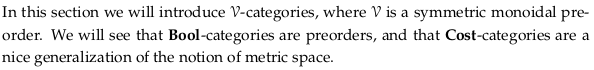## 2.3.1. 𝓥-categories#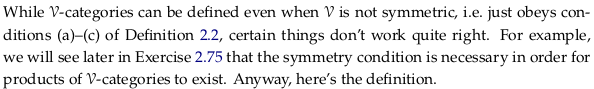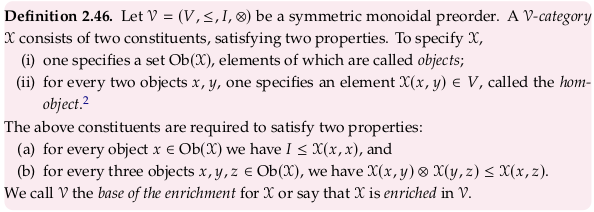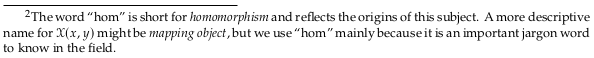A 𝓥-category is a generalization of a category, see Enriched category. However, the definition given in this section is not of an enriched category (as Wikipedia gives it); see section 4.4.4. for that definition.

In the context of Chp. 2 we can define “the” hom-object (in the language of Definition 2.46, defining a 𝓥-category) as a boolean or real number. In a Set-category (a regular category) we could say “the” hom-object is a set and hence we call it a hom-set. When we say “the” hom-element (in the language of Definition 2.79, defining a quantale) we are talking about $$v ⊸ w$$, which is only the same as the hom-object if the category is self-enriched (see Remark 2.89).

With either understanding you can see enrichment as labeling the edges of a graph; the hom-object is the label. However, this labelling has to be done in a way that respects e.g. the triangle equality.

You should usually read 𝓥-category as “monoidal category” rather than “V category” audibly, only because it is more semantically meaningful. The author actually defines it to mean “symmetric monoidal category” in Chp. 2; and in addition only deals with the special case of preorders.

To remember part (b) of this definition at this point, you should really think of it in terms of Bool: if x ≤ y and y ≤ x, then x ≤ z. In Chp. 4 we’ll see how it effectively defines composition.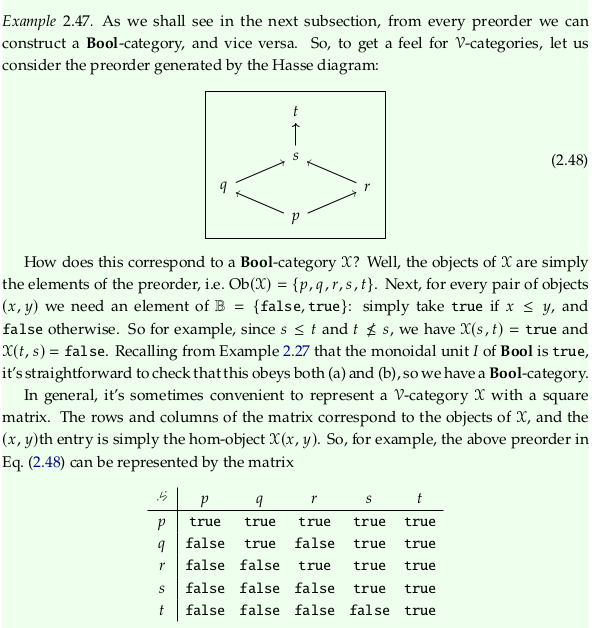## 2.3.2. Preorders as Bool-categories#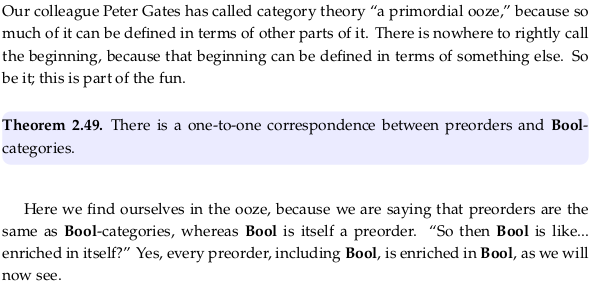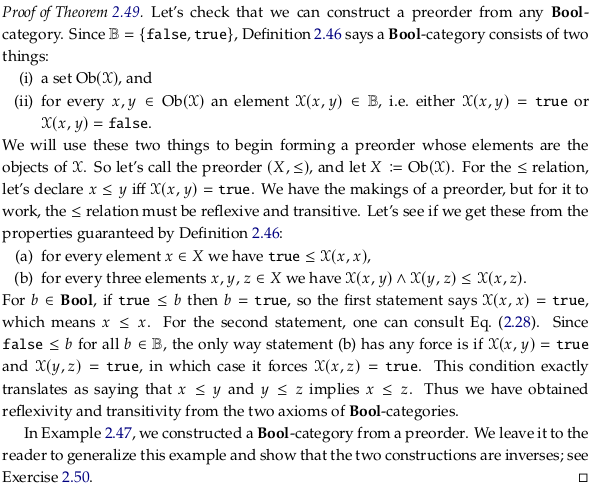### Exercise 2.50.#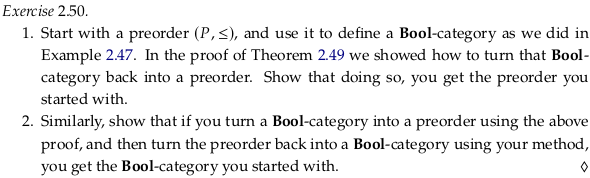To answer 1., the objects of $$\cat{X}$$ are simply the elements of the preorder, i.e. $$Ob(\cat{X}) = P$$. For every pair of objects $$(x, y)$$ we need an element of $$\BB = \{false, true\}$$. As before, simply take $$true$$ if $$x \leq y$$ and $$false$$ otherwise to define the hom-object $$\cat{X}(x, y)$$.

To go backwards as in Theorem 2.49, let $$P := Ob(\cat{X})$$. For the $$\leq$$ relation, let’s declare $$x \leq y$$ iff $$\cat{X}(x, y) = true$$. We’ve clearly gotten back to where we started.

To answer 2., let $$X := Ob(\cat{X})$$. For the $$\leq$$ relation, let’s declare $$x \leq y$$ iff $$\cat{X}(x, y) = true$$.

To go back to a $$\Bool$$-category, the objects of $$\cat{X}$$ are simply the elements of the preorder, i.e. $$Ob(\cat{X}) = X$$. For every pair of objects $$(x, y)$$ we need an element of $$\BB = \{false, true\}$$. As before, simply take $$true$$ if $$x \leq y$$ and $$false$$ otherwise to define the hom-object $$\cat{X}(x, y)$$. We’ve clearly gotten back to where we started.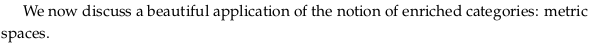## 2.3.3. Lawvere metric spaces#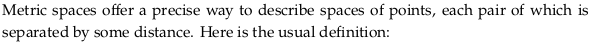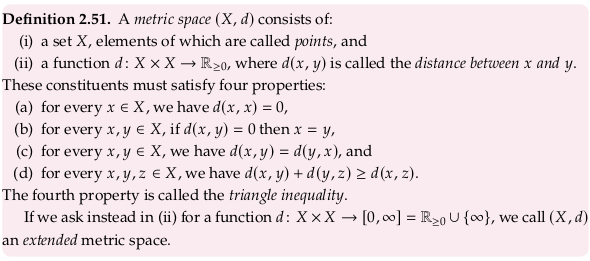See also Pseudoquasimetrics. Dropping (b) in Definition 2.51 is equivalent to adding the “pseudo” prefix and dropping (c) is equivalent to adding the “quasi” prefix.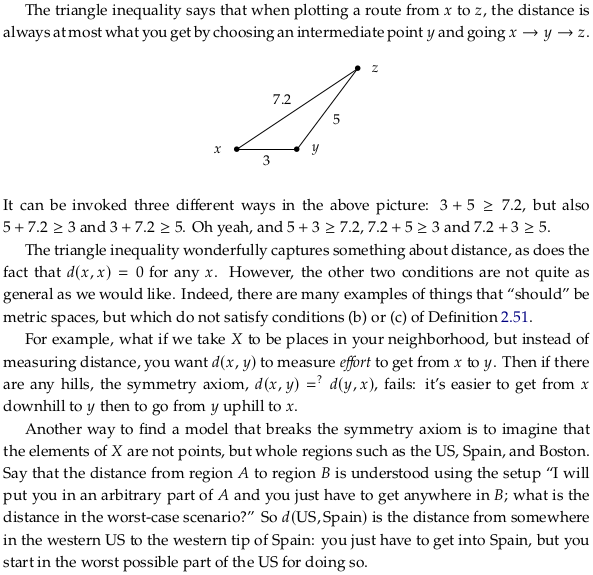### Exercise 2.52.#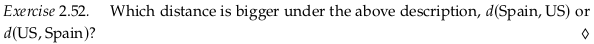The distance $$d(\mathrm{US}, \mathrm{Spain})$$? is greater because the US is much larger, and so the worst case scenario (the far side of the country) is has a greater distance to cover in the starting country.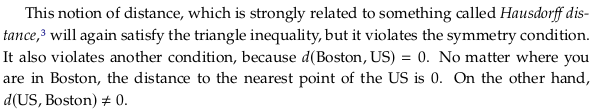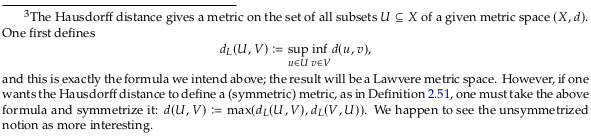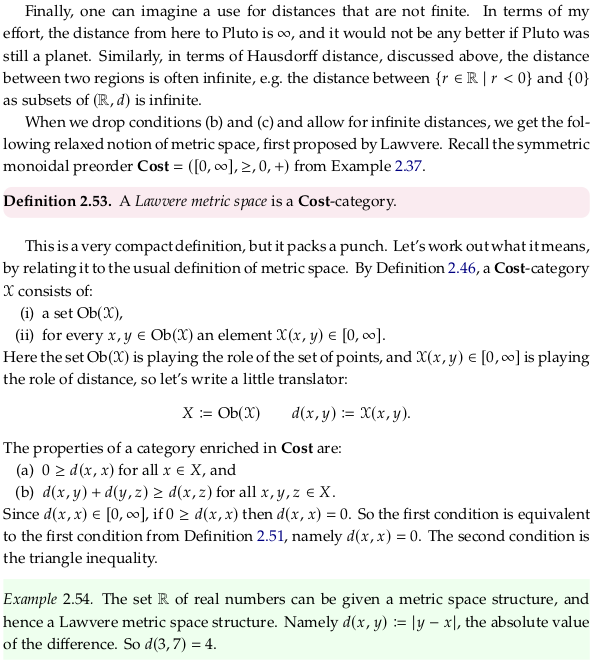### Exercise 2.55.#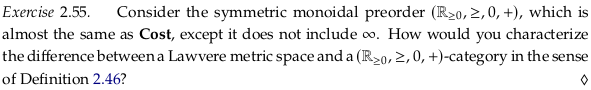The set of objects doesn’t include $$\infty$$, and the hom-object also can’t map $$\infty$$ to the distance between any two objects/elements. The distances in a space of this type could not be infinite.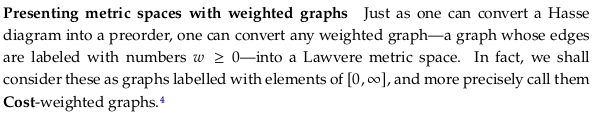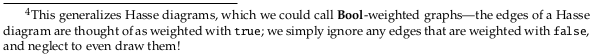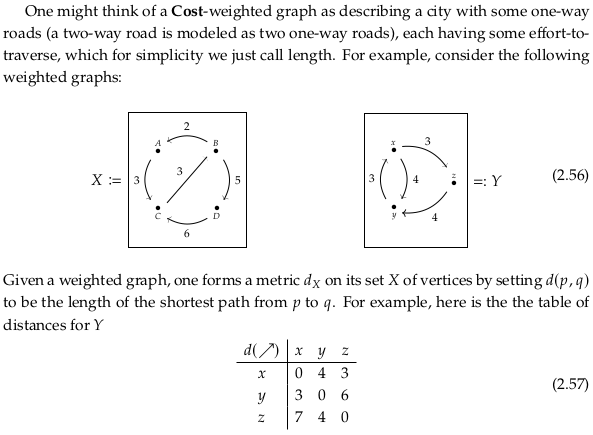### Exercise 2.58.#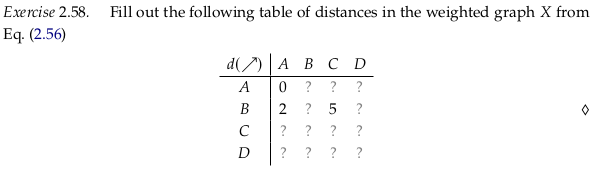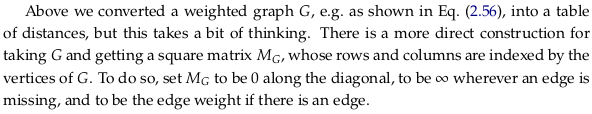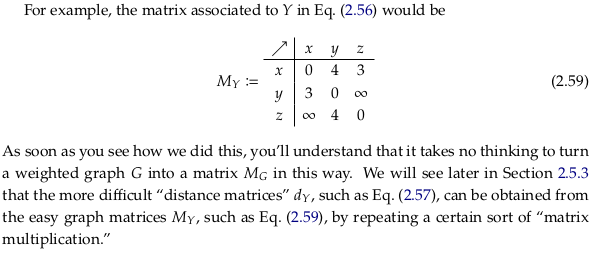### Exercise 2.60.#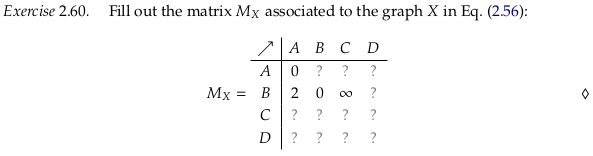## 2.3.4. 𝓥-variations on preorders and metric spaces#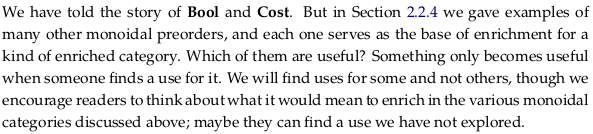import pandas as pd

def prove_v_category(df, mon_prod, preorder_rel):
for row in df.index:
for col in df.columns:
for idx in range(0,len(df.index)):
a = mon_prod(df.iloc[row, idx], df.iloc[idx, col])
assert preorder_rel(a, df.iloc[row,col]), (row,idx,col)


### Exercise 2.61.#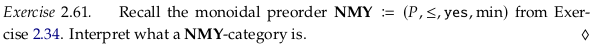Consider the following graph:Where we interpret:

• Dashed lines to mean “Maybe”

• Solid lines to mean “Yes”

• Missing lines to mean “No”

This graph corresponds to the following matrix:One way to interpret this is as a preorder that allows for uncertainty in whether two items are related. While we are confident that C ≤ B, and that A ≰ B, we are making no claims about whether $$C ≤_? A$$ and $$B ≤_? A$$.

For a similar interpretation, see the example based on the totally ordered set {0,½,1} in Heyting algebra § Examples. In a Heyting algebra we some have true and false claims, but we leave room for saying we “don’t know” whether some things are implied by another. In this case, we leave room for only one intermediate level of uncertainty (maybe).

When you are trying to communicate your uncertainty on a subject to someone else, this suggests a way to do so. Write down all the propositions you are considering as objects, perhaps with a coding table mapping e.g. the variable $$A$$ to some proposition. Then draw arrows in shades of gray, where a black arrow indicates you know something to be so and a missing arrow indicates you believe that the source of the arrow never implies the target of the arrow. You may discover that you only disagree on the shades of gray of the arrows.

The first property requires that the diagonal be “Yes” (as we see above):

$I = yes \leq n(x,x)$

Checking the second property by brute force:

diag_val = 1.0
mdf = pd.DataFrame(
index=range(3), columns=range(3),
data=[[diag_val, 0.0, 0.0], [0.5, diag_val, 0.0],[0.5, 1.0, diag_val]])
display(mdf)
prove_v_category(mdf, min, lambda x,y: x <= y)

0 1 2
0 1.0 0.0 0.0
1 0.5 1.0 0.0
2 0.5 1.0 1.0

To get a little more intuitive feel, consider the second required property of an $$\mathcal{M}$$-category:

$min(x≤y,y≤z) ≤ (x≤z)$

For paths that are only two edges long, you can interpret this as instructions to take the minimum of the two paths. For longer paths, you can see this as considering all possible intermediates y between x and z where y must be precomputed. For this to be true for all intermediates (all y) we must take the maximum of all the paths/options.

As discussed in Exercise 2.34, we could also have constructed the NMY monoidal preorder from max:

$max(x≤y,y≤z) ≤ (x≤z)$

A similar table for NMY built from max is much less interesting because any non-zero relationship anywhere forces non-zero relationships everywhere:

diag_val = 1.0
mdf = pd.DataFrame(
index=range(3), columns=range(3),
data=[[diag_val, 1.0, 1.0], [1.0, diag_val, 1.0],[1.0, 1.0, diag_val]])
display(mdf)
prove_v_category(mdf, max, lambda x,y: x <= y)

0 1 2
0 1.0 1.0 1.0
1 1.0 1.0 1.0
2 1.0 1.0 1.0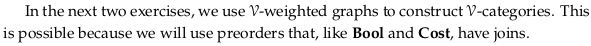### Exercise 2.62.#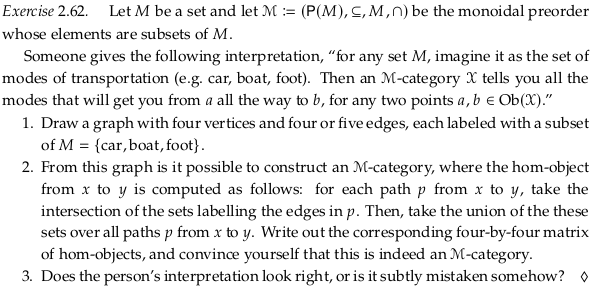The first property requires that the diagonal be {car, boat, foot}:

$I = M = \{car, boat, foot\} \subseteq n(x,x)$

We can check the matrix is an $$\mathcal{M}$$-category by brute force:

cbf = set([0,1,2])
bf = set([1,2])
f = set()
e = set()
sdf = pd.DataFrame(
index=range(4), columns=range(4),
data=[[cbf,cbf,f,f],[e,cbf,e,e],[e,e,cbf,e],[e,f,bf,cbf]]
)
display(sdf)
prove_v_category(sdf, set.intersection, lambda x,y: x <= y)

0 1 2 3
0 {0, 1, 2} {0, 1, 2} {2} {2}
1 {} {0, 1, 2} {} {}
2 {} {} {0, 1, 2} {}
3 {} {2} {1, 2} {0, 1, 2}

To get a little more intuitive feel, consider the second required property of an $$\mathcal{M}$$-category:

$n(x,y) ∩ n(y,z) \subseteq n(x,z)$

For paths that are only two edges long, you can interpret this as the instructions to:

take the intersection of the sets labelling the edges in p.

For longer paths, you can see this as considering all possible intermediates y between x and z where y must be precomputed. For this to be true for all intermediates (all y) we must:

take the union of these sets over all paths p from x to y.

Larger sets indicate two nodes are “more connected” but in a boolean sense for every element in the set. You could also use a graph like this to communicate multiple ways to solve a problem.

### Exercise 2.63.#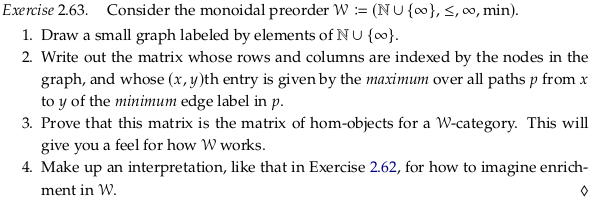The first property requires that the diagonal be ∞:

$I = ∞ \leq n(x,x)$

Checking the second property by brute force:

inf = float("inf")
wdf = pd.DataFrame(
index=range(3), columns=range(3),
data=[[inf,8,7],[0,inf,0],[0,inf,inf]]
)
display(wdf)
prove_v_category(sdf, min, lambda x,y: x <= y)

0 1 2
0 inf 8.0 7.0
1 0.0 inf 0.0
2 0.0 inf inf

The required second property (with min replaced by ∧):

$n(x,y) ∧ n(y,z) \leq n(x,z)$

For paths that are only two edges long, you can interpret this as the instructions to take the:

minimum edge label in p.

For longer paths, you can see this as considering all possible intermediates y between x and z where y must be precomputed. For this to be true for all intermediates (all y) we must take the:

maximum over all paths p

You could see enrichment in $$\mathcal{W}$$ as the opposite of enrichment in Cost. In this scenario, higher numbers mean two elements are actually more rather than less connected. The NMY and Bool enrichments could be seen as special cases of this kind of enrichment, with only three and two levels of connectedness respectively (in contrast to an infinite number of levels).

If you saw the graph as editable (as in a neural network) or at least “editable” in the sense you can turn off connections by setting them to zero, then you could see these levels of connectedness as network weights. In some design contexts (e.g. Transformer models) the opposite concept (distance) is used, and the network structure is optimized to minimize distance between key nodes. One could use the number of edges as a distance as well (assign each a value of one) if network weights haven’t been assigned yet.